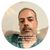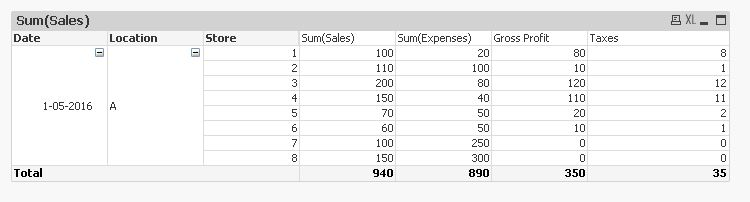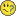QlikView App Dev

Discussion Board for collaboration related to QlikView App Development.

Announcements
QlikWorld 2022, LIVE in Denver CO., May 16-19, 2022. REGISTER NOW TO RECEIVE EARLY BIRD PRICING
cancel
Showing results for
Did you mean:Not applicable

Remove 0 value from calculation in subtotal field expressed in Pivot Table

Hi I am having a challenge removing subtotal field from a Pivot table.

For example my data is expressed in the following format

Current Output

Date                    Location              Sales              Expenses              Taxable Amount        Tax Charged (0.25 * Taxable Amt)

A                    20                        100                            0                                  0

1st May 2016              B                  100                        80                            20                                  5

C                  120                      100                            20                                  5

Total                    240                      280                          -40                                  -69.75

Desired Output

Date                    Location              Sales              Expenses              Taxable Amount        Tax Charged (0.25 * Taxable Amt)

A                    20                        100                            0                                  0

1st May 2016              B                  100                        80                            20                                  5

C                  120                      100                            20                                  5

Total                    240                      280                          40                                  10

Issues

As evident, the main issue is that my data for the column named Taxable amount is expressed as a calculated expression

if((Sum (Sales)-Sum ([Expenses])) < 0, 0, (Sum (Sales)-Sum ([Expenses])))

It achieved my desired output for the taxable amount and taxes. However the main issue arises when I expressed the subtotal in a Pivot Table. I do not wish to include values that are 0 in the calculation of the subtotal.

Can anyone advise me on how to achieve the desired output expressed in pivot table?

1 Solution

Accepted SolutionsMVP

This works as required I think -9 RepliesPartner

Hi,

Can you send me sample qvw file?

Muthukumar PandiyanSpecialist III

Hi TanHong,

For taxable amount:

If(Dimensionality() < 2,

Fabs(Sum(Sales)-Sum(Expenses)),

Sum(Sales)-Sum(Expenses))

For tax amount:

If(Dimensionality() < 2,

Fabs(Sum(Sales)-Sum(Expenses)),

Sum(Sales)-Sum(Expenses))*0.25

Regards!!Specialist

Hi Tan,

Couldo be this possibility?

Regards

Miguel del ValleMVP

Check this -Not applicable
Author

thank you all for the help here. Unfortunately it did not solve my issue.

My guess is perhaps my questions was poorly formatted resulting in the confusion.I am only interested in calculating the subtotal using the value calculated in my GP and Taxes's expressions. I have tried aggre() and dimensionality() but it appears those solutions are not exactly what I am looking for.

The main reasons is because Qlikview take into considerations of my calculated negative gross profit value instead of using 0 as stated in my GP expressionsMVP

This works as required I think -MVP

Gross Profit exp -

Sum(Aggr(if(Sum(Sales)-Sum(Expenses)<0,0,Sum(Sales)-Sum(Expenses)),Date,Location,Store))

Taxes -

Sum(Aggr(if(0.10*(Sum(Sales)-Sum(Expenses))<0,0,0.10*(Sum(Sales)-Sum(Expenses))),Date,Location,Store))Partner

You can simplify the expressions like this:

Taxable:

Sum(Aggr(RangeMax(0, Sum(Sales) - Sum(Expenses)), Date, Location, Store))

Tax:

Sum(Aggr(RangeMax(0, Sum(Sales) - Sum(Expenses)), Date, Location, Store)) * 0.25

Logic will get you from a to b. Imagination will take you everywhere. - A EinsteinNot applicable
Author

It worked! Thank you Qlikview Community! I learnt something new along the way. Much appreciatedCommunity Browser